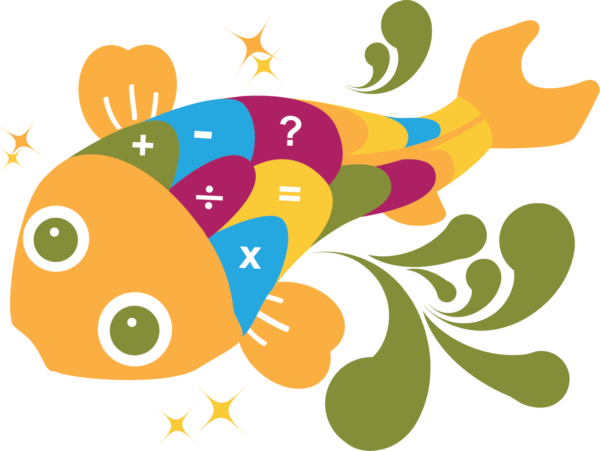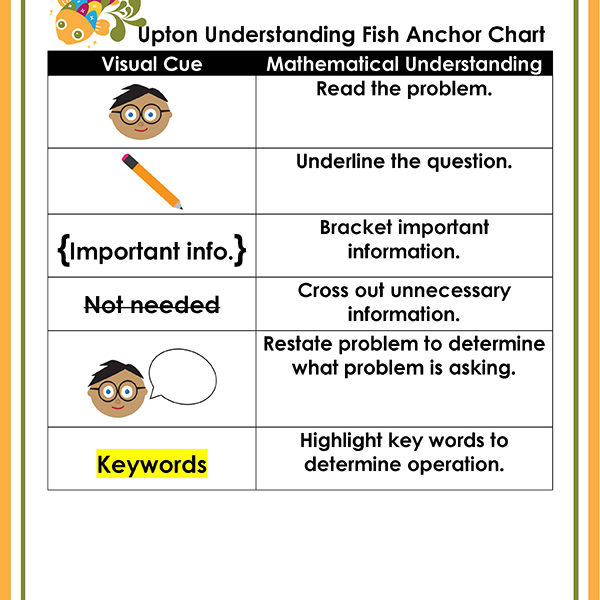# Debuting Upton Understanding Fish

May 11th 2014Upton Understanding Fish here, and problem-solving starts with me.
Practice with me at Problem-Solving Pond,
And your math skills will increase here and beyond.

Upton is the newest strategy animal in our Problem-Solving Pond, a CCSS Math Strategy Unit created to help teachers overcome Common Core math challenges and employ problem-solving strategies with confidence and fidelity. Read on to learn more about Upton and download Upton’s complete unit here.

WHAT is mathematical understanding?  Mathematical understanding means that students understand the story problem and follow the problem-solving steps. Mathematical understanding includes many steps: identify question; identify key information; get rid of erroneous information; determine the operation; solve using an appropriate, efficient strategy. Students who have a firm mathematical understanding should also be able to explain the problem-solving steps they used and justify their solution using an additional strategy.

WHY is mathematical understanding important? Understanding story problems can be very challenging because it requires multi-step, higher-level thinking processes. Students are required to process several pieces of information before starting any mathematical operations. If students do not understand the problem, they will solve it incorrectly, even if they have a strong repertoire of strategies.  It is critical that students learn how to divide the problem into smaller chunks to thoroughly understand each component.

HOW do I teach mathematical understanding?  Since mathematical understanding involves so many steps, teachers should teach each step explicitly and introduce the next step after proficiency is demonstrated. This allows the teacher to isolate individual steps first and then gradually integrate the steps together. This unit breaks each component of mathematical understanding into mini-lessons and accompanying practice pages.  Students learn how to:

1. Read the problem and underline the question.
2. Bracket important information and cross out unnecessary information.
3. Restate the problem to determine what is being asked to solve.
4. Use key words to choose an operation.

This unit uses a spiral review format so each mathematical understanding lesson builds upon previous lessons. This ensures that students get ample practice of these critical skills.

Each component of mathematical understanding has a visual cue included in the practice pages. An accompanying Upton Understanding Fish Anchor Chart is included in the unit (see below).WHEN should I introduce mathematical understanding?  Mathematical understanding is the foundation for problem-solving and must be introduced before any mathematical operations are performed. Mathematical understanding should be modeled and practiced all year long in the context of meaningful story problems.

• Incorporate multi-sensory activities.  Allow students to act out the problem, use manipulatives and/or highlight key information in the problem. My class has their own Upton Understanding Fish, a small, inexpensive stuffed animal that I purchased from Ebay.During problem-solving time, I have students sit at a circle at the carpet area and together we analyze the problem. First I read the problem while students follow along on a paper copy and then I toss Upton to a student to read the question. That student tosses Upton to another student who identifies important information and then tosses to another student who determines if there is any unnecessary information. Upton is tossed for a final time to determine key words and operation used to solve the problem. The students absolutely love this activity and it promotes engagement and academic conversations. Often students will argue different points and learn to use the story problem to justify their positions.